Click to Chat

1800-1023-196

+91-120-4616500

CART 0

• 0

MY CART (5)

Use Coupon: CART20 and get 20% off on all online Study Material

ITEM
DETAILS
MRP
DISCOUNT
FINAL PRICE
Total Price: Rs.

There are no items in this cart.
Continue Shopping```Introduction

Table of Content

Units for Measurement

Fundamental Units

Derived Unit

Units and Dimensional Analysis: Conversion of Units

Matter

Classification of Matter

Elements

Compound

Mixture

Chemistry is the science of substances, their properties, structures and their transformation.
As all objects in the universe are made of matter. Chemistry is the branch of the science which deals with the study of material object.
Study of chemistry is very interesting which covers various aspects of our culture and environment. All development in any science are based on scientific approach as in chemistry too. In order to achieve correct results, one has to rely upon the various skills connected with the measurements of quantities during a physical or chemical change. The degree of accuracy is closely linked with precision of the measuring instrument as well as on the skill of the person engaged in measurement.

Physical Property: The property which can be measured without changing the chemical composition of the substance is known as physical property like mass, volume, density, refractive index etc.

Chemical Property: The property which can be evaluated at the cost of matter itself is known as chemical property. For example combustible nature of hydrogen gas can be verified by burning of hydrogen. The sweet taste of sugar by consuming it.

Units for Measurement

All physical quantities have to be measured. The value of a physical quantity is expressed as the product of the numerical value and the unit in which it is expressed.

Fundamental Units

Fundamental units are those units which can neither be derived from one another nor they can be further resolved into any other units.
The seven fundamental units of measurement in S.I. system.

Quantity

Name of unit

Abbreviation

Mass

Kilogram

Kg

Length

Meter

m

Temperature

Kelvin

K

Amount of substance

Mole

Mol

Time

Second

S

Electric current

Ampere

A

Luminous intensity

Candela

Cd

Derived Unit

Some quantities are expressed as a function of more than one fundamental units known as derived units. For example velocity, acceleration, work, energy etc.

Quantity with Symbol

Unit (S.I.)

Symbol

Velocity (v)

Metre per sec

ms-1

Area (A)

Square metre

m2­

Volume (V)

Cubic metre

m3

Density (r)

Kilogram m-3

Kg m-3

Energy (E)

Joule (J)

Kg m2s-2

Force (F)

Newton (N)

Kg ms-2

Frequency (n)

Hertz

Cycle per sec

Pressure (P)

Pascal (Pa)

Nm-2

Electrical charge

Coulomb (C)

A-s (ampere – second)

Units and Dimensional Analysis: Conversion of Units

The simplest way to carry out calculations that involve different units is to use dimensional analysis. In this method a quantity expressed in one unit is converted into an equivalent quantity with a different unit by using conversion factor which express the relationship between units:

Original quantity  x  conversion factor = equivalent quantity

(in former unit)                                         (in other unit)

This is based on the fact that ratio of each fundamental quantity in one unit with their equivalent quantity in other unit is equal to one For example in case of massSo 1 kg = 2.205 pond = 1000 gm

In this way any derived unit first expressed in dimension and each fundamental quantities like mass length time are converted in other system of desired unit to work out the conversion factor.

Example 1:

Question:

How unit of work / energy i.e. joule, in S.I. system is related with unit erg in C.G.S system

Solution:

Dimension of work = force x displacement = MLT-2´ L = ML2T-2

1 joule = 1 kg (1 metre)2´ (1see)-2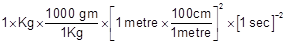100 gm x (100)2 x1 em2 x (1 sec)-2

1000x10000 x 1 gm x1 cm2 x1 sec-1

1 joule = 107 erg

Similarly we can deduce other conversion factor for other quantity in different unit by the dimensional analysis method

Another interesting example is the conversion of litre – atmosphere to joule (the SI unit of energy) by multiplying with two successive unit factors. Thus,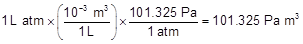Knowing that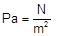, we can writeHence 1 L atm = 101.325 J

Example 2:

Question:

What is the mass of 1 L of mercury in grams and in kilograms if the density of liquid mercury is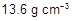?

Solution:

We know the relationship,and

Also,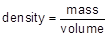We can write, mass = (volume) (density)

Therefore, the mass of 1 L of mercury is equal to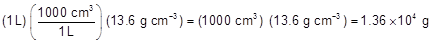The mass in kilograms can be calculated as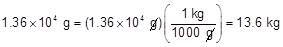(Remember,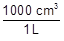andare conversion factors with which we have to multiply for getting our answer in appropriate units).

Matter

Anything that exhibits inertia is known as matter.  The quantity of matter is its mass. e.g. chalk table.

Classification of Matter

This classification of matter is based upon chemical composition of various substances. According to this matter can be further divided into two types, pure substance and mixture. Mixtures are also of two types, homogenous mixtures and heterogeneous mixtures.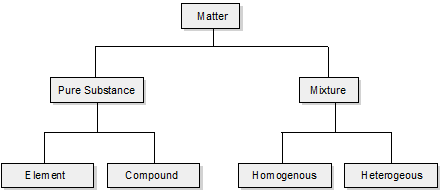Elements

The primary stuff present in all the substance is known as element, whose smallest unit is known as atom. Total 112 elements are known till date of which 92 are naturally occurring elements rest are results of artificial transmutation. There are 88 metals, 18 non metals and 6 metaloids.

Compound

A non elemental pure substance is called a compound in which more than one atom of elements are linked by chemical bonds formed due to chemical reaction. The resulting molecule is a electrically neutral particle of constant continuous composition.

Mixture

Mixtures are the aggregate of more than one type of pure substance whose chemical identity remains maintained even in mixtures. Their constituent ratio may vary unlike compound.
For example – sugar + water = sugar syrup, Gun-powder 75 % KNO3 10% sulphur + 15% carbon
There are two types of mixture (a) homogeneous (b) heterogenous

Homogeneous mixtures are those whose composition for each part remains constant. For example aqueous and gaseous solution.

Heterogeneous mixtures are those whose composition may vary for each and every part. For example soil, concrete mixtures.

```### Course Features

• 731 Video Lectures
• Revision Notes
• Previous Year Papers
• Mind Map
• Study Planner
• NCERT Solutions
• Discussion Forum
• Test paper with Video Solution